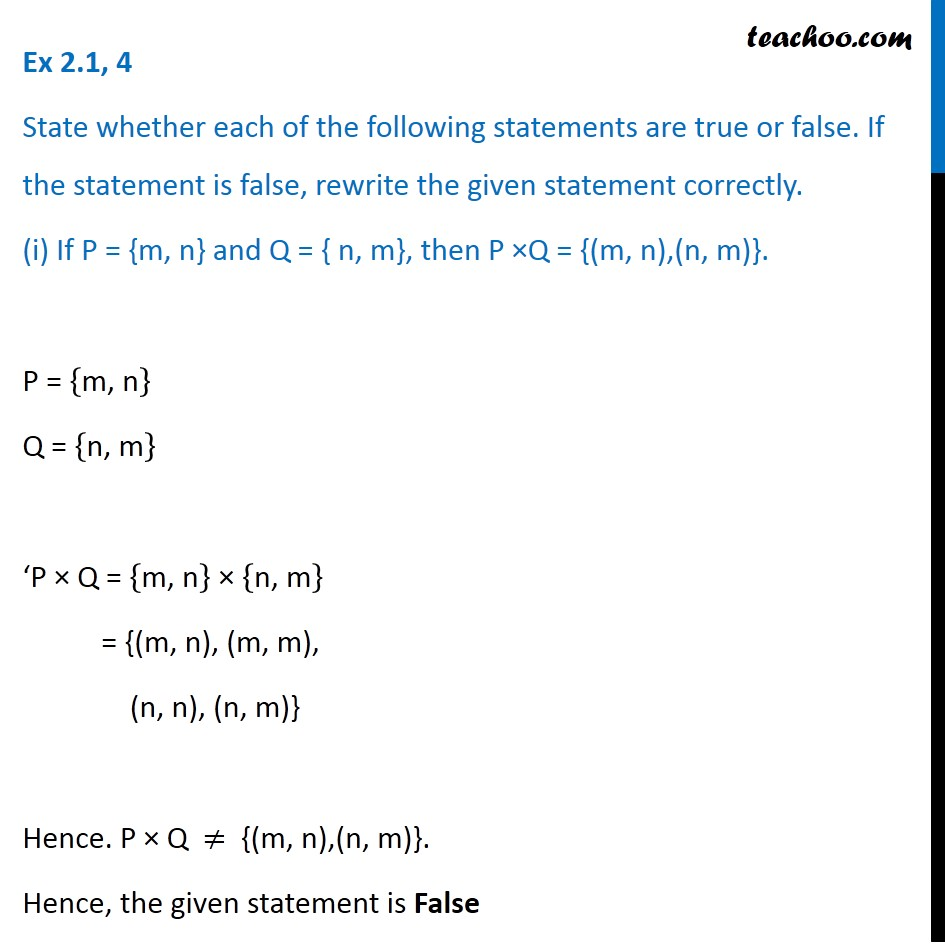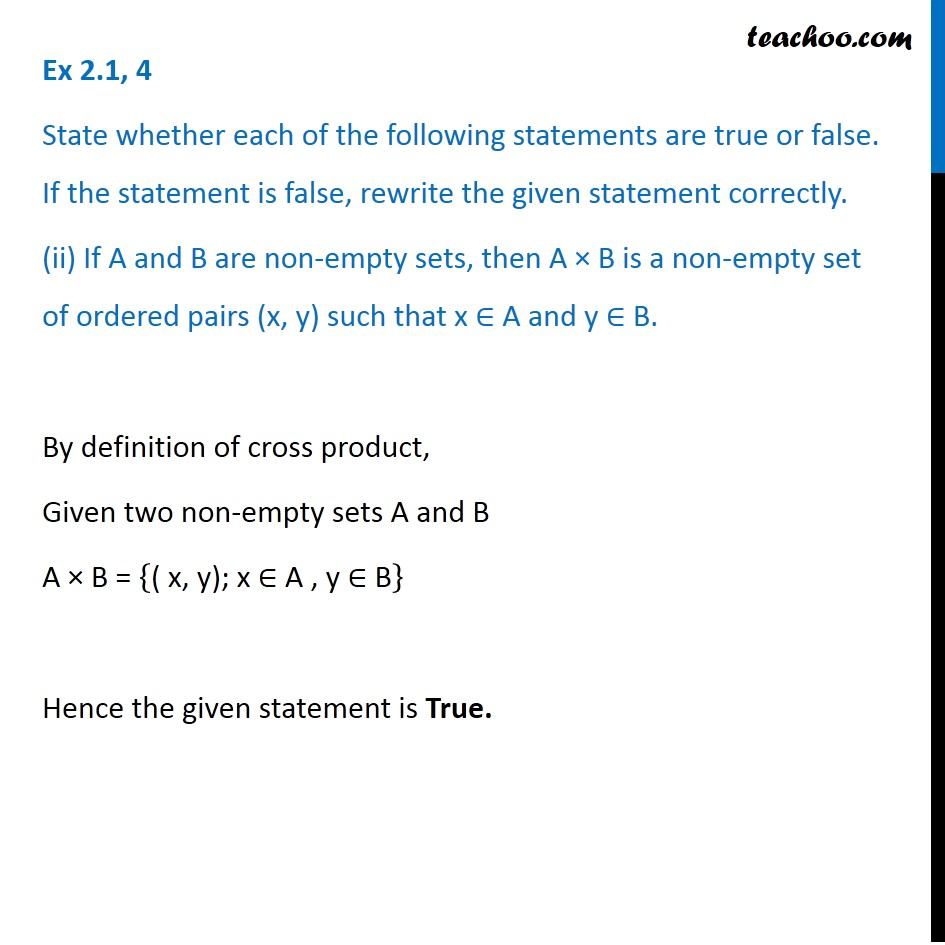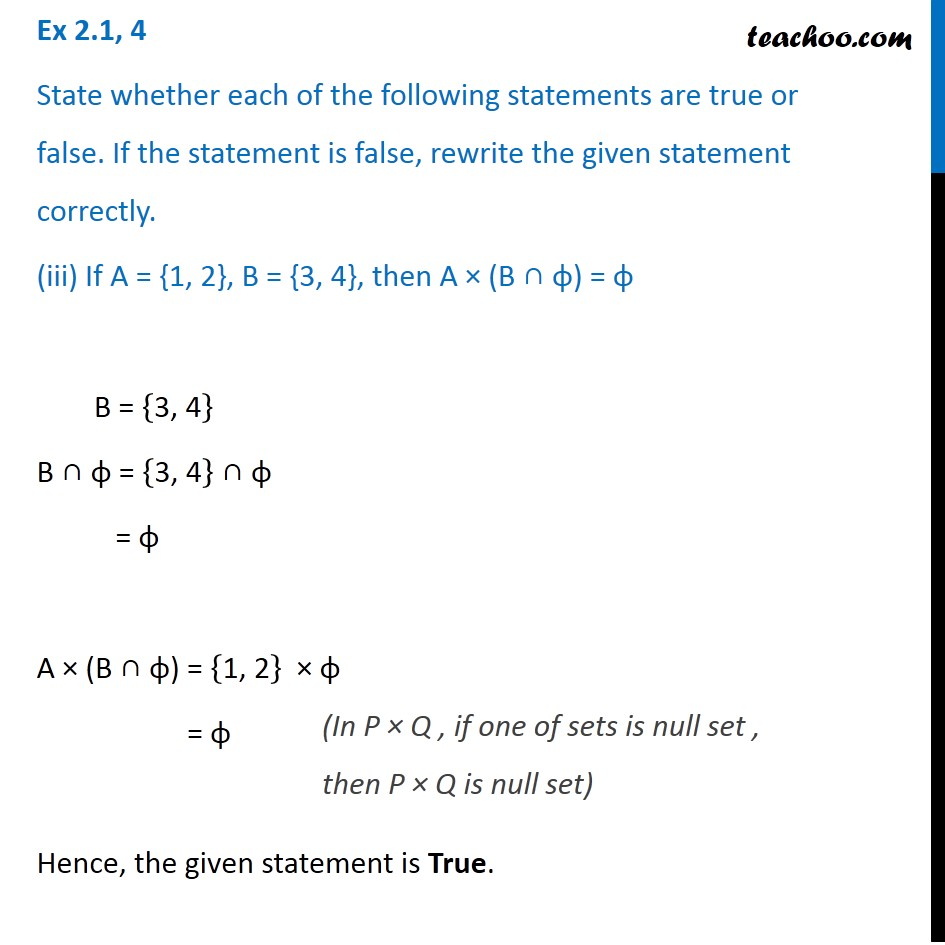Learn All Concepts of Chapter 2 Class 11 Relations and Function - FREE. Check - Relation and Function Class 11 - All Concepts1. Chapter 2 Class 11 Relations and Functions
2. Serial order wise
3. Ex 2.1

Transcript

Ex 2.1, 4 State whether each of the following statements are true or false. If the statement is false, rewrite the given statement correctly. (i) If P = {m, n} and Q = { n, m}, then P ×Q = {(m, n),(n, m)}. P = {"m, n" } Q = {"n, m" } ‘P × Q = {"m, n" } × {"n, m" } = {(m, n), (m, m), (n, n), (n, m)} Hence. P × Q ≠ {(m, n),(n, m)}. Hence, the given statement is False The correct statement is If P = {m, n} and Q = { n, m}, then P × Q = {(m, n), (m, m), (n, n), (n, m)} Ex 2.1, 4 State whether each of the following statements are true or false. If the statement is false, rewrite the given statement correctly. (ii) If A and B are non-empty sets, then A × B is a non-empty set of ordered pairs (x, y) such that x ∈ A and y ∈ B. By definition of cross product, Given two non-empty sets A and B A × B = {"( x, y); x ∈ A , y ∈ B" } Hence the given statement is True. Ex 2.1, 4 State whether each of the following statements are true or false. If the statement is false, rewrite the given statement correctly. (iii) If A = {1, 2}, B = {3, 4}, then A × (B ∩ φ) = φ B = {"3, 4" } B ∩ φ = {"3, 4" } ∩ φ = φ A × (B ∩ φ) = {"1, 2" } × φ = φ Hence, the given statement is True. (In P × Q , if one of sets is null set , then P × Q is null set)

Ex 2.1